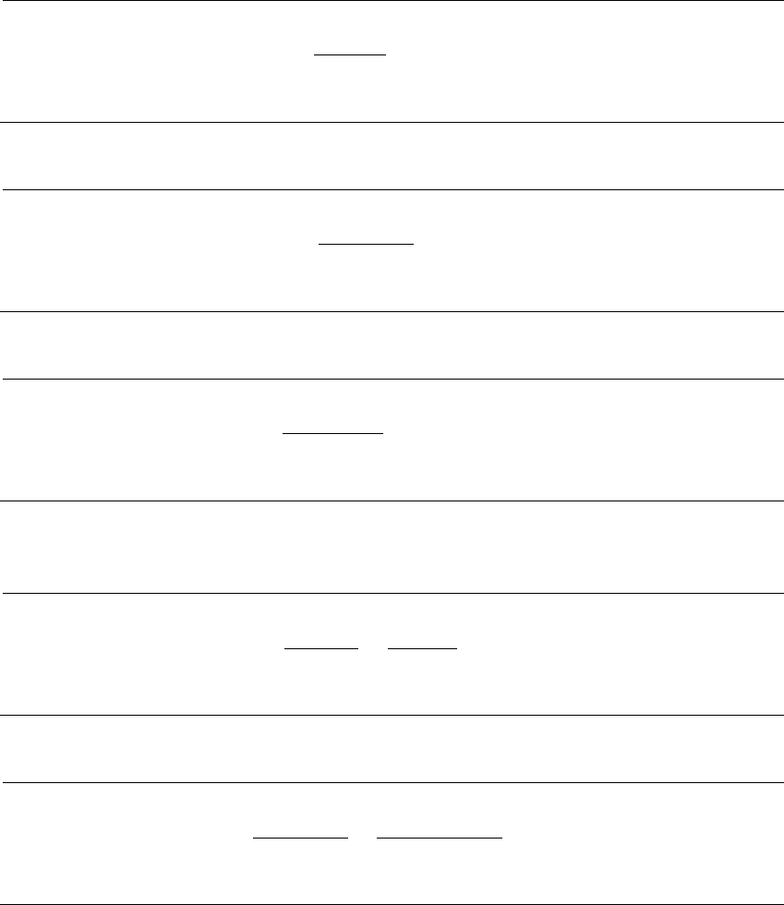Study Guides (400,000)
US (230,000)
UCSD (1,000)
ECON (100)
Midterm

# ECON 132 Midterm: Practice Questions+Solutions for Midterm #1Exam

Department
Economics
Course Code
ECON 132
Professor
Richard Carson
Study Guide
Midterm

This preview shows pages 1-2. to view the full 8 pages of the document.Econ 132 - Problem Set 1 Solution - Winter 2019
1 Conversions
a. How many British Thermal Units (BTUs) are equivalent to 5 kilowatt hours (5 kWh)
of electricity?
5kWh ×3412Btu
kWh =17,060 Btu
b. How many million British Thermal Units (MMBTUs) are in 2 short tons of coal?
2short ton ×25 MMBtu
short ton =50 MMBtu
c. Six blue barrels of oil (6bbl) contain how many million British Thermal Units (MMBTUs)?
6bbl ×5.8MMBtu
bbl =34.8 MMBtu
d. How many blue barrels (bbl) of oil are equivalent to 2 million cubic feet (2MMcf)
of natural gas?
2MMcf ×1000Mcf
1MMcf ×0.178bbl
Mcf =356 bbl
e. Four short-tons of coal are equivalent are equivalent to how many Mcf of natural gas?
4short ton ×25 MMBtu
1short ton ×1Mcf
1.035MMBTU =96.6 Mcf
1

Only pages 1-2 are available for preview. Some parts have been intentionally blurred.2 Thermal Eﬃciency
a. The Indian River power plant in Florida uses 8,000 BTU of energy to generate each kilo-
watt hour of electricity. Find its thermal eﬃciency
3,412 Btu/kWh
8,000 Btu/kWh = 0.4265 42.65%
3 Elasticities
a. (arc elasticity) Compute the arc elasticity of a consumer who uses 40 gallons of gas per
week when the price is \$3.50 per gallon and 50 gallons per week when the price is
\$3 per gallon.
ǫarc =Q2Q1
0.5(Q1+Q2)P2P1
0.5(P1+P2)
=50 40
0.5(50 + 40) 33.50
0.5(3 + 3.50)
=1.44
2

Unlock to view full version

Only pages 1-2 are available for preview. Some parts have been intentionally blurred.b. (point elasticity) Find the point elasticity of demand for a consumer whose demand for
gasoline is given by the function Q= 80 4P0.2P2when the price is \$4 per gallon.
ǫpoint =
dQ
Q
dp
p
=dQ
dp ×p
Q
p= \$4
Q(p) = 80 4p0.2p2
Q(4) = 80 440.216
= 60.8
dQ
dp =40.4p
=5.6
ǫpoint =5.64
60.8
=0.368
c. I consume 50 gallons of gas and 15 gallons of diesel per month and I make \$2000 per month.
The price of gas is \$4 per gallon and the price of diesel is \$5 per gallon, If the price of diesel
falls to \$4 per gallon and I consume 40 gallons of gas, what is my cross price elasticity of
demand for gasoline with respect to the price of diesel?
ǫQGPD=QG2QG1
0.5(QG2+QG1)PD2PD1
0.5(PD2+PD1)
=40 50
0.5(40 + 50)45
0.5(4 + 5)
= 1
3